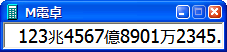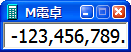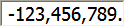Calculator software that can enlarge characters even in a compact (date / time calculation, also corresponds to a scientific calculator)Various settings, options, date / time calculation etc. enter from the menu right click on the main window.

### Features

• The display of the button can be erased and it is compact. [Options]-[Display]
Windows standard calculator has a numeric keypad button. However, in fact, I look at the numeric keypad on the keyboard and enter. Buttons on the screen are not necessary. Since M-Calc can be set only to "numerical display", the area will be smaller. You can also delete the title bar. Also, since you can change the font, you can enlarge the letters. It has enough function as a calculator.

• It works in the same way as a real calculator (correspondence between ordinary calculator and function calculator) [option]-[calculation method]-[input method]
It corresponds to three types of input method: ordinary calculator (Sharp, Citizen type), function calculator without mathematical expression display, and function calculator with mathematical expression display.

• Date and time calculation
• Loan repayment amount calculation
• Unit conversion
• Triangulation calculation (even if you are not familiar with trigonometric functions, you can handle them)